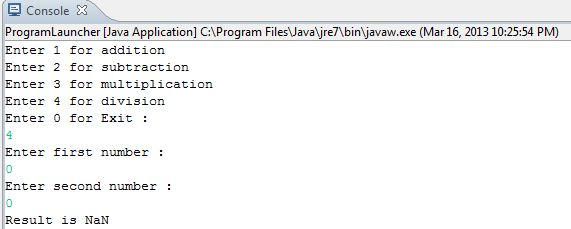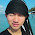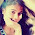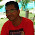## Saturday, 16 March 2013

### Basic Calculator program in Java

I guess we are ready to write some good quality basic Java programs. One thing I would like to state here is that even if you are finding Java a bit new, you will eventually master it only by practice and hence it is essential to keep writing codes and not just understanding the theory.

Let write a very basic Java program for calculator. There is no GUI involved since we have not covered Swing yet. This program will just take two numbers as input and perform the required operations like addition, subtraction etc.  Though it is basic it will cover most of the topics we have learned till now. I am writing and running Java programs on Eclipse and hence you may find it's snapshots here but you can even write codes and execute using the command line or use other IDE's like Netbeans. So let's get started.

#### Update - 3rd July 2018

You can find the latest code for basic calculator program in my GitHub Gist -

Code and working is explained in following youtube video -

#### Java code for Calculator

Lets first create a class where we implement the calculator logic. Create a new Java project in Eclipse called Calculator. Next, create a package in it called basicCalculator. In this package make two new classes
class Calculation where we would implement the calculator logic and class ProgramLauncher which will have the main() function from which we will create class Calculation object and execute it.

Create class Calculation and insert the code below.

```package basicCalculator;

import java.util.Scanner;

public class Calculation {

private float firstOperannd;
private float secondOperannd;
private float result;
private int operator;
private Scanner input = new Scanner(System.in);
private boolean exitCalculator = false;

public void startCalculator() {
while (!exitCalculator) {

+ "Enter 2 for subtraction \n"
+ "Enter 3 for multiplication \n"
+ "Enter 4 for division \n" + "Enter 0 for Exit : ");
operator = input.nextInt();

switch (operator) {
case 1:
System.out.println("Result is " + result);
break;

case 2:
result = subtract();
System.out.println("Result is " + result);
break;

case 3:
result = multiply();
System.out.println("Result is " + result);
break;

case 4:
result = divide();
System.out.println("Result is " + result);
break;

case 0:
exitCalculator = true;
System.out.println("Calculator program Terminated \n");
break;

default:

}
}
}

System.out.println("Enter first number : ");
firstOperannd = input.nextInt();
System.out.println("Enter second number : ");
secondOperannd = input.nextInt();
return firstOperannd + secondOperannd;
}

private float subtract() {
System.out.println("Enter first number : ");
firstOperannd = input.nextInt();
System.out.println("Enter second number : ");
secondOperannd = input.nextInt();
return firstOperannd - secondOperannd;
}

private float multiply() {
System.out.println("Enter first number : ");
firstOperannd = input.nextInt();
System.out.println("Enter second number : ");
secondOperannd = input.nextInt();
return firstOperannd * secondOperannd;
}

private float divide() {
System.out.println("Enter first number : ");
firstOperannd = input.nextInt();
System.out.println("Enter second number : ");
secondOperannd = input.nextInt();
return firstOperannd / secondOperannd;
}

}
```
There are many points that need to be explained here so be patient. First let's get our code up and running.
So create next class ProgramLauncher and insert the code below.

```package basicCalculator;

public class ProgramLauncher {

public static void main(String[] args) {

Calculation calculator = new Calculation();
calculator.startCalculator();

}

}
```

Just execute the program and try to check the output for various test cases. For how to create a project, package, class, how to see the standard output in eclipse please go through our post on Hello World.

When you execute this program you will see output something like below -

It clearly says what you need to input and what are the corresponding operations. If you press 0, the program will terminate. Go ahead and try out various cases. More importantly, try division by 0 or something like 0/0. I will explain these but you can go ahead and try it now.

#### Understanding the Code

Launcher code is very simple. All we do is create a Calculation class object and call the startCalculator() method. Just for the record, we could call the method like calculator.startCalculator(); because the method is defined to be public. You can't do the same with other functions like add() as they are private. We will explain why we have taken such a decision but till then understand the program behavior.

Let's go to our calculator logic code now.

#### Initializations

We have defined two float operands firstOperannd and secondOperand.Similarly we have defined float result to store the result, int operator to store values corresponding to various operations and finally a scanner object to take user input. Again note that all these are instance variables and are private. So at any point of time, you cannot access them using class Calculation object. They can only be manipulated using public functions provided. We also have exitCalculator to keep track of whether to exit our program or not. The default value is set to false which means do not close the program unless user says so.

#### startCalculator() function

Inside this function, we have a while loop which keeps on executing unless exitCalculator is set to true.
Inside the loop, we ask the user to choose which operation he is interested in. He must input one of the available options i.e 0,1,2,3 or 4. We then have the switch statement which has operator as the argument.

Note: Switch statement supports String as an argument only in JSE 7. So I have used int to make the program run for everyone but if you are using  Java SE 7 you can go ahead and use String as input directly.

Inside the switch statements, we have cases each corresponding to different operations. We have defined separate functions for each operation i.e add(), subtract() etc and all we do in each case in a switch statement is call these functions. Note the usage of the break statement. We are computing the result in each case and printing the same on standard output. Each function computes the result and returns it back. Note that if a user enters 0 then exitCalculator is set to true and the program terminates. For all other inputs default statement is executed which says you must provide correct input. Execution will continue unless user enters 0.

#### Why are functions like add() defined private?

Before you define anything in Java or for that matter any programming language you must decide what is it going to be used for. Like, in this case, we know that add() function will only be used by

startCalculator() method and we don't want to allow any usage like objectName.add(). Hence functions are defined to be private. These functions can only be used in other functions of the same class.

#### Interesting cases

Let's discuss some of the interesting cases that might arise in case of division.
• 4/0: What happens when I divide any number by 0. Technically you can't divide by 0 and you must get an error. You will get an error if you divide by zero in the case of integers but in case of float, you will get the result as infinity. Note if you are using your firstOperand and secondOperand as int's you need to handle that divide by 0 runtime exception. Also if you use int and user types in something like 4.2 it will be truncated to 4.
• 0/0: What happens when you divide 0 by a 0. Definitely not infinity in this case. It's a value called  NaN(not a number). Again if you use int you will get a runtime exception and program will terminate. These are some of the reasons why we have chosen float over int.

#### Eclipse output snapshotsOutput for 6/0 operationOutput for 0/0 operation

Do post any doubts you have in the comments sections.

1.thank you very much this really help me :D

2.this is not simple classes program

3.GUI is possible ?
thanks

4.Thanks so much for this help...
It's very interesting

5.Im having error at the calculator.startCalculator(); im still a beginner and this our project.

1.@Archana what error are you getting?

t> UA-39527780-1